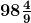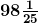# The overall width in cm of several

Question:

The overall width in cm of several wide-screen televisions are 97.28 cm,cm,cm and 97.94 cm. Express these numbers as rational numbers in the form p/q and arrange the widths in ascending order.

Solution:

From the question,

The overall width in cm of several wide screen television are,

97.28 cm = 9728/100 … [∵by the decimal removing method]

By dividing both numerator and denominator by 4 we get,

= 2432/25cmcm = by converting mixed fraction into improper fraction we get,

= 886/9 cmcm = by converting mixed fraction into improper fraction we get,

= 2451/25 cm

97.94 cm = 9794/100 … [∵by the decimal removing method]

By dividing both numerator and denominator by 2 we get,

= 4897/50cm

Now, we have to take the LCM of denominators to arrange them in ascending order.

The LCM of the denominators 25, 9, 25 and 50 is 450

∴ 2432/25= [(2432×18)/ (25×18)] = (43776/450)

(886/9) = [(886×50)/ (9×50)] = (44300/450)

(2451/25) = [(2451×18)/ (25×18)] = (44118/450)

(4897/50) = [(4897×9)/ (50×9)] = (44073/450)

Then,

Now, 43776 < 44073 < 44118 < 44300

Hence, in ascending order = (2432/25) < (4897/50) < (2451/25) < (886/9)

∴97.28 < 97.94 <cm <cm